# Slide rule facts for kids

Kids Encyclopedia Facts

The slide rule, or slipstick, is a mechanical analog computer. The slide rule is used mainly for multiplication and division, and also for "scientific" functions such as roots, logarithms and trigonometry, but usually not for addition or subtraction.

There are many different styles of slide rules. They are usually linear or circular. They have a standardised set of markings (called scales). These scales are used for mathematical computations. Some slide rules have been made for special use, as for aviation or finance. Those slide rules have special scales for those applications, as well as normal scales.

William Oughtred and others developed the slide rule in the 1600s. The slide rule is based on the work on logarithms by John Napier. Before electronic calculators were developed, slide rules were the tool used most often in science and engineering. The use of slide rules continued to grow through the 1950s and 1960s even as digital computing devices were gradually introduced; but around 1974 the pocket calculator made the slide rule largely obsolete and most suppliers left the business.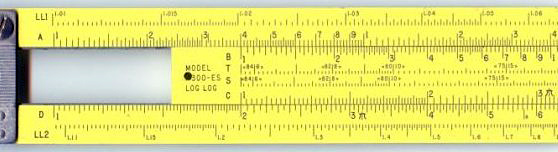A slide rule positioned so as to multiply by 2. Each number on the D (bottom) scale is double the number above it on the C (middle) scale.

## Basic concepts

In its most basic form, the slide rule uses two logarithmic scales to allow rapid multiplication and division of numbers. These common operations can be time-consuming and error-prone when done on paper. More complex slide rules allow other calculations, such as square roots, exponentials, logarithms, and trigonometric functions.

Mathematical calculations are done by aligning a mark on the sliding central strip with one on one of the fixed strips. The relative position of other marks can then be observed. Numbers aligned with the marks give the approximate value of the product, quotient, or other calculated result.

The user determines the location of the decimal point in the result, based on mental estimation. Scientific notation is used to track the decimal point in more formal calculations. Addition and subtraction steps in a calculation are generally done mentally or on paper, not on the slide rule.

Most slide rules have three linear strips of the same length. The strips are aligned in parallel and interlocked so that the central strip can be moved lengthwise relative to the other two. The outer two strips are fixed so that their relative positions do not change.

Some slide rules ("duplex" models) have scales on both sides of the rule and slide strip, others on one side of the outer strips and both sides of the slide strip, still others on one side only ("simplex" rules). A sliding cursor with a vertical alignment line is used to find corresponding points on scales that are not next to each other or, in duplex models, are on the other side of the rule. The cursor can also record an intermediate result on any of the scales.

## Using a slide rule for calculation

### Multiplication

A logarithm transforms the operations of multiplication and division to addition and subtraction according to the rules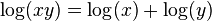$\log(xy) = \log(x) + \log(y)$ and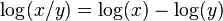$\log(x/y) = \log(x) - \log(y)$. Moving the top scale to the right by a distance of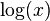$\log(x)$, by matching the beginning of the top scale with the label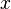$x$ on the bottom, aligns each number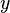$y$, at position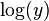$\log(y)$ on the top scale, with the number at position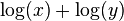$\log(x) + \log(y)$ on the bottom scale. Because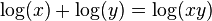$\log(x) + \log(y) = \log(xy)$, this position on the bottom scale gives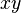$xy$, the product of$x$ and$y$. For example, to calculate 3*2, the 1 on the top scale is moved to the 2 on the bottom scale. The answer, 6, is read off the bottom scale where 3 is on the top scale. In general, the 1 on the top is moved to a factor on the bottom, and the answer is read off the bottom where the other factor is on the top.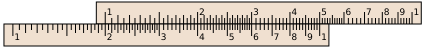Operations may go "off the scale;" for example, the diagram above shows that the slide rule has not positioned the 7 on the upper scale above any number on the lower scale, so it does not give any answer for 2×7. In such cases, the user may slide the upper scale to the left until its right index aligns with the 2, effectively multiplying by 0.2 instead of by 2, as in the illustration below: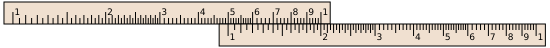Here the user of the slide rule must remember to adjust the decimal point appropriately to correct the final answer. We wanted to find 2×7, but instead we calculated 0.2×7=1.4. So the true answer is not 1.4 but 14. Resetting the slide is not the only way to handle multiplications that would result in off-scale results, such as 2×7; some other methods are:

• (1) Use the double-decade scales A and B.
• (2) Use the folded scales. In this example, set the left 1 of C opposite the 2 of D. Move the cursor to 7 on CF, and read the result from DF.
• (3) Use the CI inverted scale. Position the 7 on the CI scale above the 2 on the D scale, and then read the result off of the D scale, below the 1 on the CI scale. Since 1 occurs in two places on the CI scale, one of them will always be on-scale.
• (4) Use both the CI inverted scale and the C scale. Line up the 2 of CI with the 1 of D, and read the result from D, below the 7 on the C scale.

Method 1 is easy to understand, but entails a loss of precision. Method 3 has the advantage that it only involves two scales.

### Division

The illustration below demonstrates the computation of 5.5/2. The 2 on the top scale is placed over the 5.5 on the bottom scale. The 1 on the top scale lies above the quotient, 2.75. There is more than one method for doing division, but the method presented here has the advantage that the final result cannot be off-scale, because one has a choice of using the 1 at either end.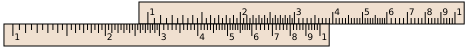### Other operations

In addition to the logarithmic scales, some slide rules have other mathematical functions encoded on other auxiliary scales. The most popular were trigonometric, usually sine and tangent, common logarithm (log10) (for taking the log of a value on a multiplier scale), natural logarithm (ln) and exponential (ex) scales. Some rules include a Pythagorean scale, to figure sides of triangles, and a scale to figure circles. Others feature scales for calculating hyperbolic functions. On linear rules, the scales and their labeling are highly standardized, with variation usually occurring only in terms of which scales are included and in what order:

 A, B two-decade logarithmic scales, used for finding square roots and squares of numbers C, D single-decade logarithmic scales K three-decade logarithmic scale, used for finding cube roots and cubes of numbers CF, DF "folded" versions of the C and D scales that start from π rather than from unity; these are convenient in two cases. First when the user guesses a product will be close to 10 but is not sure whether it will be slightly less or slightly more than 10, the folded scales avoid the possibility of going off the scale. Second, by making the start π rather than the square root of 10, multiplying or dividing by π (as is common in science and engineering formulas) is simplified. CI, DI, DIF "inverted" scales, running from right to left, used to simplify 1/x steps S used for finding sines and cosines on the D scale T used for finding tangents and cotangents on the D and DI scales ST, SRT used for sines and tangents of small angles and degree–radian conversion L a linear scale, used along with the C and D scales for finding base-10 logarithms and powers of 10 LLn a set of log-log scales, used for finding logarithms and exponentials of numbers Ln a linear scale, used along with the C and D scales for finding natural (base e) logarithms and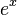$e^x$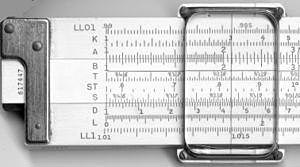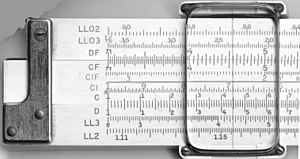The scales on the front and back of a K&E 4081-3 slide rule.

The Binary Slide Rule manufactured by Gilson in 1931 performed an addition and subtraction function limited to fractions.

#### Roots and powers

There are single-decade (C and D), double-decade (A and B), and triple-decade (K) scales. To compute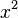$x^2$, for example, locate x on the D scale and read its square on the A scale. Inverting this process allows square roots to be found, and similarly for the powers 3, 1/3, 2/3, and 3/2. Care must be taken when the base, x, is found in more than one place on its scale. For instance, there are two nines on the A scale; to find the square root of nine, use the first one; the second one gives the square root of 90.

For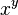$x^y$ problems, use the LL scales. When several LL scales are present, use the one with x on it. First, align the leftmost 1 on the C scale with x on the LL scale. Then, find y on the C scale and go down to the LL scale with x on it. That scale will indicate the answer. If y is "off the scale," locate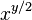$x^{y/2}$ and square it using the A and B scales as described above.

#### Trigonometry

The S, T, and ST scales are used for trigonometric functions and multiples of trig functions, for angles in degrees. Many slide rules have their S, T, and ST scales marked with degrees and minutes. So-called decitrig models use decimal fractions of degrees instead.

#### Logarithms and exponentials

Base-10 logarithms and exponentials are found using the L scale, which is linear. Some slide rules have a Ln scale, which is for base e.

The Ln scale was invented by an 11th grade student, Stephen B. Cohen, in 1958. The original intent was to allow the user to select an exponent x (in the range 0 to 2.3) on the Ln scale and read ex on the C (or D) scale and ex on the CI (or DI) scale. Pickett, Inc. was given exclusive rights to the scale. Later, the inventor created a set of "marks" on the Ln scale to extend the range beyond the 2.3 limit, but Pickett never incorporated these marks on any of its slide rules.

Slide rules are not typically used for addition and subtraction, but it is nevertheless possible to do so using two different techniques.

The first method to perform addition and subtraction on the C and D (or any comparable scales) requires converting the problem into one of division. For addition, the quotient of the two variables plus one times the divisor equals their sum: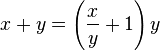$x + y = \left(\frac{x}{y} + 1\right) y$

For subtraction, the quotient of the two variables minus one times the divisor equals their difference: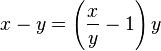$x - y = \left(\frac{x}{y} - 1\right) y$

This method is similar to the addition/subtraction technique used for high-speed electronic circuits with the logarithmic number system in specialized computer applications like the Gravity Pipe (GRAPE) supercomputer and hidden Markov models.

The second method uses a sliding linear L scale available on some models. Addition and subtraction are performed by sliding the cursor left (for subtraction) or right (for addition) then returning the slide to 0 to read the result.

## Physical design

### Standard linear rules

The length of the slide rule is quoted in terms of the nominal length of the scales. Scales on the most common "10-inch" models are actually 25 cm in length, as they were made to metric standards, though some rules offer slightly extended scales to simplify manipulation when a result overflowed. Pocket rules are typically 5 inches. Models a couple of meters long were sold to be hung in classrooms for teaching purposes. 

Typically the divisions mark a scale to a precision of two significant figures, and the user estimates the third figure. Some high-end slide rules have magnifying cursors that make the markings easier to see. Such cursors can effectively double the accuracy of readings, permitting a 10-inch slide rule to serve as well as a 20-inch.

Various other conveniences have been developed. Trigonometric scales are sometimes dual-labeled, in black and red, with complementary angles, the so-called "Darmstadt" style. Duplex slide rules often duplicate some of the scales on the back. Scales are often "split" to get higher accuracy.

Specialized slide rules were invented for various forms of engineering, business and banking. These often had common calculations directly expressed as special scales, for example loan calculations, optimal purchase quantities, or particular engineering equations. For example, the Fisher Controls company distributed a customized slide rule adapted to solving the equations used for selecting the proper size of industrial flow control valves.

### Circular slide rules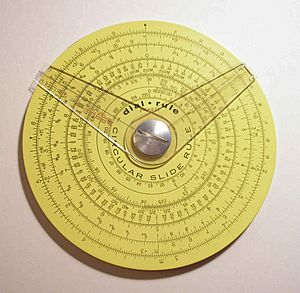Pickett circular slide rule with two cursors. (4.25 in./10.9 cm diameter) Reverse has additional scale and one cursor.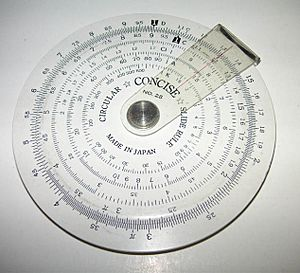A simple circular slide rule, made by Concise Co., Ltd., Tokyo, Japan, with only inverse, square and cubic scales. On the reverse is a handy list of 38 metric/imperial conversion factors.

Circular slide rules come in two basic types, one with two cursors (left), and another with a movable disk and a single cursor (right). The dual cursor versions perform multiplication and division by maintaining a fixed angle between the cursors as they are rotated around the dial. The single cursor version operates more like the standard slide rule through the appropriate alignment of the scales.

The basic advantage of a circular slide rule is that the longest dimension of the tool was reduced by a factor of about 3 (i.e. by π). For example, the outer scale of a 10 cm circular would have a maximum precision equal to a 30 cm ordinary slide rule. Circular slide rules also eliminate "off-scale" calculations, because the scales were designed to "wrap around"; they never have to be re-oriented when results are near 1.0—the rule is always on scale. However, for non-cyclical non-spiral scales such as S, T, and LL's, the scale length is shortened to make room for end margins.

Circular slide rules are mechanically more rugged and smoother-moving, but their scale alignment precision is sensitive to the centering of a central pivot; a minute 0.1 mm off-centre of the pivot can result in a 0.2 mm worst case alignment error. The pivot, however, does prevent scratching of the face and cursors. The highest accuracy scales are placed on the outer rings. Rather than "split" scales, high-end circular rules use spiral scales for more complex operations like log-of-log scales. One eight-inch premium circular rule had a 50-inch spiral log-log scale.

The main disadvantages of circular slide rules are the difficulty in locating figures along a rotating disc, and limited number of scales. Another drawback of circular slide rules is that less-important scales are closer to the center, and have lower precisions. Most students learned slide rule use on the linear slide rules, and did not find reason to switch.

One slide rule remaining in daily use around the world is the E6B. This is a circular slide rule first created in the 1930s for aircraft pilots to help with dead reckoning. With the aid of scales printed on the frame it also helps with such miscellaneous tasks as converting time, distance, speed, and temperature values, compass errors, and calculating fuel use. The so-called "prayer wheel" is still available in flight shops, and remains widely used. While GPS has reduced the use of dead reckoning for aerial navigation, and handheld calculators have taken over many of its functions, the E6B remains widely used as a primary or backup device and the majority of flight schools demand that their students have some degree of its mastery.

In 1952, Swiss watch company Breitling introduced a pilot's wristwatch with an integrated circular slide rule specialized for flight calculations: the Breitling Navitimer. The Navitimer circular rule, referred to by Breitling as a "navigation computer", featured airspeed, rate/time of climb/descent, flight time, distance, and fuel consumption functions, as well as kilometer–nautical mile and gallonliter fuel amount conversion functions.

### Materials

Traditionally slide rules were made out of hard wood such as mahogany or boxwood with cursors of glass and metal. At least one high precision instrument was made of steel.

In 1895, a Japanese firm, Hemmi, started to make slide rules from bamboo, which had the advantages of being dimensionally stable, strong and naturally self-lubricating. These bamboo slide rules were introduced in Sweden in September, 1933 , and probably only a little earlier in Germany. Scales were made of celluloid or plastic. Later slide rules were made of plastic, or aluminum painted with plastic. Later cursors were acrylics or polycarbonates sliding on Teflon bearings.

All premium slide rules had numbers and scales engraved, and then filled with paint or other resin. Painted or imprinted slide rules were viewed as inferior because the markings could wear off. Nevertheless, Pickett, probably America's most successful slide rule company, made all printed scales. Premium slide rules included clever catches so the rule would not fall apart by accident, and bumpers to protect the scales and cursor from rubbing on tabletops. The recommended cleaning method for engraved markings is to scrub lightly with steel-wool. For painted slide rules, and the faint of heart, use diluted commercial window-cleaning fluid and a soft cloth.

## History

The slide rule was invented around 1620–1630, shortly after John Napier's publication of the concept of the logarithm. Edmund Gunter of Oxford developed a calculating device with a single logarithmic scale, which, with additional measuring tools, could be used to multiply and divide. The first description of this scale was published in Paris in 1624 by Edmund Wingate (c. 1593 – 1656), an English mathematician, in a book entitled “L'usage de la reigle de proportion en l'arithmetique & geometrie”. The book contains a double scale on one side of which is a logarithmic scale and on the other a tabular scale. In 1630, William Oughtred of Cambridge invented a circular slide rule, and in 1632 he combined two Gunter rules, held together with the hands, to make a device that is recognizably the modern slide rule. Like his contemporary at Cambridge, Isaac Newton, Oughtred taught his ideas privately to his students, but delayed in publishing them, and like Newton, he became involved in a vitriolic controversy over priority, with his one-time student Richard Delamain and the prior claims of Wingate. Oughtred's ideas were only made public in publications of his student William Forster in 1632 and 1653.

In 1677, Henry Coggeshall created a two-foot folding rule for timber measure, called the Coggeshall slide rule. His design and uses for the tool gave the slide rule purpose outside of mathematical inquiry.

In 1722, Warner introduced the two- and three-decade scales, and in 1755 Everard included an inverted scale; a slide rule containing all of these scales is usually known as a "polyphase" rule.

In 1815, Peter Roget invented the log log slide rule, which included a scale displaying the logarithm of the logarithm. This allowed the user to directly perform calculations involving roots and exponents. This was especially useful for fractional powers.

### Modern form

The more modern form was created in 1859 by French artillery lieutenant Amédée Mannheim, "who was fortunate in having his rule made by a firm of national reputation and in having it adopted by the French Artillery." It was around that time, as engineering became a recognized professional activity, that slide rules came into wide use in Europe. They did not become common in the United States until 1881, when Edwin Thacher introduced a cylindrical rule there. The duplex rule was invented by William Cox in 1891, and was produced by Keuffel and Esser Co. of New York.

Astronomical work also required fine computations, and in 19th century Germany a steel slide rule about 2 meters long was used at one observatory. It had a microscope attached, giving it accuracy to six decimal places.

In World War II, bombardiers and navigators who required quick calculations often used specialized slide rules. One office of the U.S. Navy actually designed a generic slide rule "chassis" with an aluminum body and plastic cursor into which celluloid cards (printed on both sides) could be placed for special calculations. The process was invented to calculate range, fuel use and altitude for aircraft, and then adapted to many other purposes.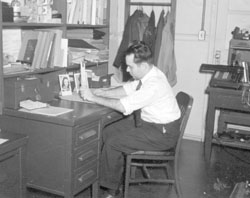Engineer using a slide rule. Note mechanical calculator in background.

Throughout the 1950s and 1960s the slide rule was the symbol of the engineer's profession (in the same way that the stethoscope symbolizes the medical profession). German rocket scientist Wernher von Braun brought two 1930s vintage Nestler slide rules with him when he moved to the U.S. after World War II to work on the American space program. Throughout his life he never used any other pocket calculating devices; slide rules served him perfectly well for making quick estimates of rocket design parameters and other figures. Aluminum Pickett-brand slide rules were carried on five Apollo space missions, including to the moon, according to advertising on Pickett's N600 slide rule boxes .

Some engineering students and engineers carried ten-inch slide rules in belt holsters, and even into the mid 1970s this was a common sight on campuses. Students also might keep a ten-or twenty-inch rule for precision work at home or the office while carrying a five-inch pocket slide rule around with them.

In 2004, education researchers David B. Sher and Dean C. Nataro conceived a new type of slide rule based on prosthaphaeresis, an algorithm for rapidly computing products that predates logarithms. There has been little practical interest in constructing one beyond the initial prototype, however. 

### Decline

The importance of the slide rule began to diminish as electronic computers, a new but very scarce resource in the 1950s, became widely available to technical workers during the 1960s. The introduction of Fortran in 1957 made computers practical for solving modest size mathematical problems. IBM introduced a series of more affordable computers, the IBM 650 (1954), IBM 1620 (1959), IBM 1130 (1965) addressed to the science and engineering market. John Kemeny's BASIC programming language (1964) made it easy for students to use computers. The DEC PDP-8 minicomputer was introduced in 1965.

Computers also changed the nature of calculation. With slide rules, there was a great emphasis on working the algebra to get expressions into the most computable form. Users of slide rules would simply approximate or drop small terms to simplify the calculation. Fortran allowed complicated formulas to be typed in from textbooks without the effort of reformulation. Numerical integration was often easier than trying to find closed form solutions for difficult problems. The young engineer asking for computer time to solve a problem that could have been done by a few swipes on the slide rule became a humorous cliché. Many computer centers had a framed slide rule hung on a wall with the note "In case of emergency, break glass."

Another step toward the replacement of slide rules with electronics was the development of electronic calculators for scientific and engineering use. The first included the Wang Laboratories LOCI-2, introduced in 1965, which used logarithms for multiplication and division and the Hewlett-Packard HP-9100, introduced in 1968. The HP-9100 had trigonometric functions (sin, cos, tan) in addition to exponentials and logarithms. It used the CORDIC (coordinate rotation digital computer) algorithm, which allows for calculation of trigonometric functions using only shift and add operations. This method facilitated the development of ever smaller scientific calculators.

The last nail in the coffin for the slide rule was the launch of pocket-sized scientific calculators, of which the 1972 Hewlett-Packard HP-35 was the first. Such calculators became known as "slide rule" calculators since they could perform most or all of the functions on a slide rule. At several hundred dollars, even this was considered expensive for most students. While professional slide rules could also be quite expensive, drug stores often sold basic plastic models for under $20 USD. But by 1975, basic four-function electronic calculators could be purchased for under$50. By 1976 the TI-30 offered a scientific calculator for under \$25. After this time, the market for slide rules dried up quickly as small scientific calculators became affordable.

• A slide rule tends to moderate the fallacy of "false precision" and significance. The typical precision available to a user of a slide rule is about three places of accuracy. This is in good correspondence with most data available for input to engineering formulas. When a modern pocket calculator is used, the precision may be displayed to seven or more decimal places, while in reality the results can never be of greater accuracy than the input data available.
• A slide rule requires a continual estimation of the order of magnitude of the results. On a slide rule 1.5 × 30 (which equals 45) will show the same result as 1,500,000 × 0.03 (which equals 45,000). It is up to the engineer to continually determine the reasonableness of the results, something that can be lost when numbers are carelessly entered into a computer program or a calculator.
• When performing a sequence of multiplications or divisions by the same number, the answer can be often determined by merely glancing at the slide rule without any manipulation. This can be especially useful when calculating percentages, e.g., for test scores, or when comparing prices, e.g., in dollars per kilogram. Multiple speed-time-distance calculations can be performed hands-free at a glance with a slide rule.
• A slide rule does not depend on electricity.
• A slide rule is an easily replicated technology. From a given example of a slide rule, more can be constructed by a competent craftsperson from rudimentary materials using non-industrial processes.
• Slide rules are highly standardized, so there is no need to relearn anything when switching to a different rule.
• Slide rules are versatile, and can be operated in situations and environments where a human user might have reduced dexterity (for example, due to requiring protective gloves). Conversely, a calculator can be difficult to operate in such situations – a slide rule is unlikely to result in an error similar to that resulting from mistakenly pressing the wrong button on a calculator.
• Slide rules can be made out of cardboard or paper. Many free charts or specialized calculating devices made out of cardboard are actually specialized linear or circular slide rules.

One advantage of using a slide rule together with an electronic calculator is that an important calculation can be checked by doing it on both; because the two instruments are so different, there is little chance of making the same mistake twice.

• Errors may arise from mechanical imprecision.
• Calculations using the slide rule are of limited precision because of their analog inputs and outputs. Conversely, because of the discrete numerical input and floating point electronic operations, even modest modern calculators have output resolutions of at least six significant figures.

## Related pages

General information, history:

How-to's:

## Images for kidsSlide rule Facts for Kids. Kiddle Encyclopedia.